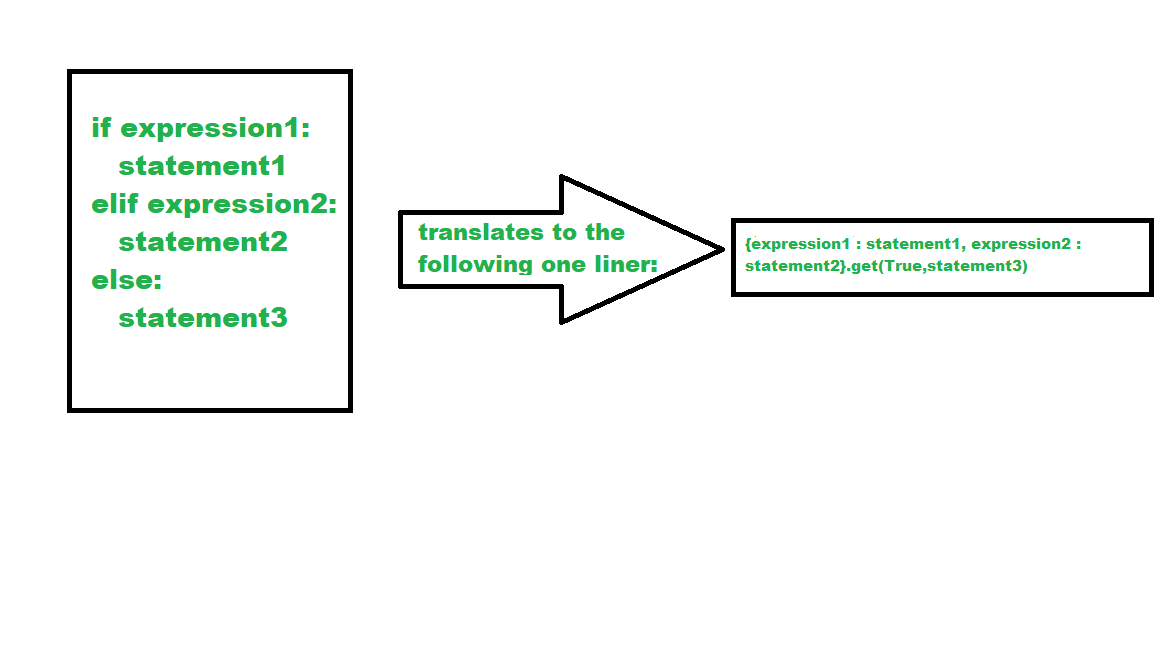# One Liner for Python if-elif-else Statements

• Last Updated : 11 Dec, 2020

If-elif-else statement is used in Python for decision-making i.e the program will evaluate test expression and will execute the remaining statements only if the given test expression turns out to be true. This allows validation for multiple expressions.

Syntax :

Attention geek! Strengthen your foundations with the Python Programming Foundation Course and learn the basics.

To begin with, your interview preparations Enhance your Data Structures concepts with the Python DS Course. And to begin with your Machine Learning Journey, join the Machine Learning - Basic Level Course

```if test expression:
Body of if

elif test expression:
Body of elif

else:
Body of else```

The concept can be implemented using the short-hand method using ternary operation.

One Liner for Python if-elif-else StatementsSyntax:

{(condition1 :  <code1>) , (condition2 :  <code2>) }.get(True, <code3>)

This can be easily interpreted as if condition 1 is true run code 1 if condition 2 is true run code 2 and if both of them are false run the third code.

Example:

## Python3

 `x ``=` `87`` ` `result ``=` `{x > ``190``: ``"First condition satisfied!"``, ``          ``x ``=``=` `87``: ``"Second condition satisfied!"``}.get(``  ``True``, ``"Third condition satisfied"``)`` ` `print``(result)`

Output:

`Second condition satisfied!`

Disclaimer: the below code won’t get you the desired results for this syntax of if-elif-else in Python:

## Python3

 `x ``=` `87`` ` `{x > ``190``: ``print``(``"First condition satisfied!"``),`` ``x ``=``=` `87``: ``print``(``"Second condition satisfied!"``)}.get(``  ``True``, ``print``(``"Third condition satisfied!"``))`

Output:

```First condition satisfied!
Second condition satisfied!
Third condition satisfied!```

My Personal Notes arrow_drop_up